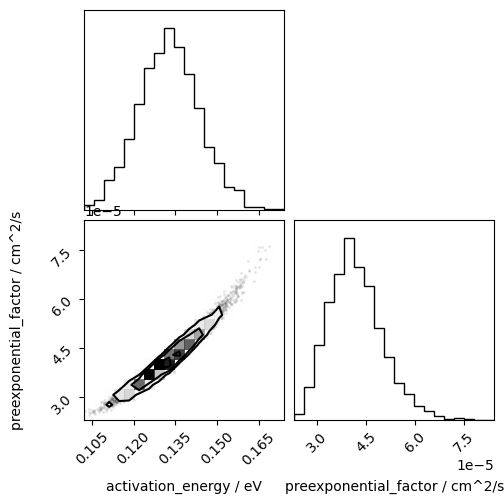# Investigation of an Arrhenius relationship#

In addition to being able to determine the mean-squared displacement and diffusion coefficient from a given simulation, kinisi also includes tools to investigate Arrhenius relationships. In this tutorial, we will look at how we can take advantage of these tools to study short, approximately 50 ps, simulations of lithium lanthanum zirconium oxide (LLZO).

Warning

The warnings that are being ignored are related to the parsing of the files by MDAnalysis and lead to unnecessary print out to the screen that we want to avoid in the web documentation.

:

import numpy as np
import MDAnalysis as mda
import matplotlib.pyplot as plt
from kinisi.analyze import DiffusionAnalyzer
from kinisi.arrhenius import StandardArrhenius
import warnings
np.random.seed(42)
warnings.filterwarnings("ignore", category=UserWarning)

/home/docs/checkouts/readthedocs.org/user_builds/kinisi/envs/latest/lib/python3.7/site-packages/tqdm/auto.py:22: TqdmWarning: IProgress not found. Please update jupyter and ipywidgets. See https://ipywidgets.readthedocs.io/en/stable/user_install.html
from .autonotebook import tqdm as notebook_tqdm


To investigate this we will loop through a series of four temperatures and append each diffusion coefficient to a list.

:

temperatures = np.array([500, 600, 700, 800])
D = []


To read these simulations we will use MDAnalysis (however, it is also possible to use data from a VASP simulation). The parser, bootstrap, and diffusion parameters are all defined for all simulations, here we only consider the diffusive regime to begin after 5 ps. Additionally, we include in the p_params a sub_sample_atoms key, this defines the sampling frequency of atoms to be used in the analysis. This facility can be particularly useful for large simulations where kinisi might encounter issues related to out-of-memory problems.

:

p_params = {'specie': 'LI',
'time_step': 5.079648e-4,
'step_skip': 100,
'min_dt': 0.001,
'sub_sample_atoms': 4,
'progress': False}
b_params = {'progress': False}
d_params = {'dt_skip': 5,
'progress': False}


File parsing and diffusion determination is then performed in a loop here.

:

for t in temperatures:
u = mda.Universe(f'_static/traj_{t}.gro', f'_static/traj_{t}.xtc')
d = DiffusionAnalyzer.from_universe(u, p_params, bootstrap_params=b_params)
d.diffusion(d_params)
D.append(d.D)

/home/docs/checkouts/readthedocs.org/user_builds/kinisi/envs/latest/lib/python3.7/site-packages/scipy/optimize/_numdiff.py:557: RuntimeWarning: invalid value encountered in subtract
df = fun(x) - f0
/home/docs/checkouts/readthedocs.org/user_builds/kinisi/envs/latest/lib/python3.7/site-packages/scipy/optimize/_numdiff.py:557: RuntimeWarning: invalid value encountered in subtract
df = fun(x) - f0


The list of diffusion coefficient objects (which are uravu.distribution.Distribution type objects) and array of temperatures can then be passed to the kinisi.arrhenius.StandardArrhenius class.

:

s = StandardArrhenius(temperatures, D)


Having created the object, we can determine the maximum likelihood values for the parameters of activation energy and the preexponential factor.

:

s.max_likelihood('diff_evo')


After determining the maximum likelihood values, we can use Markov chain Monte Carlo (MCMC) to sample the probability distributions for these.

:

s.mcmc()

100%|██████████| 1000/1000 [01:48<00:00,  9.18it/s]


We can then visualise the probability distributions for the parameters as histograms.

:

from corner import corner

:

corner(s.flatchain, labels=['$E_a$/eV', '$A$/eV'])
plt.show()It is also possible to plot these probability distributions as Arrhenius relations on the data measured values.

:

plt.errorbar(1000/s.x, s.y.n, s.y.s, marker='o', ls='', zorder=10)
for i in np.random.randint(s.activation_energy.size, size=128):
plt.plot(1000/s.x, s.function(s.x, *s.get_sample(i)), color='k', alpha=0.05)
plt.yscale('log')
plt.xlabel('$1000T^{-1}$/K$^{-1}$')
plt.ylabel('$D$/cm$^2$s$^{-1}$')
plt.show()